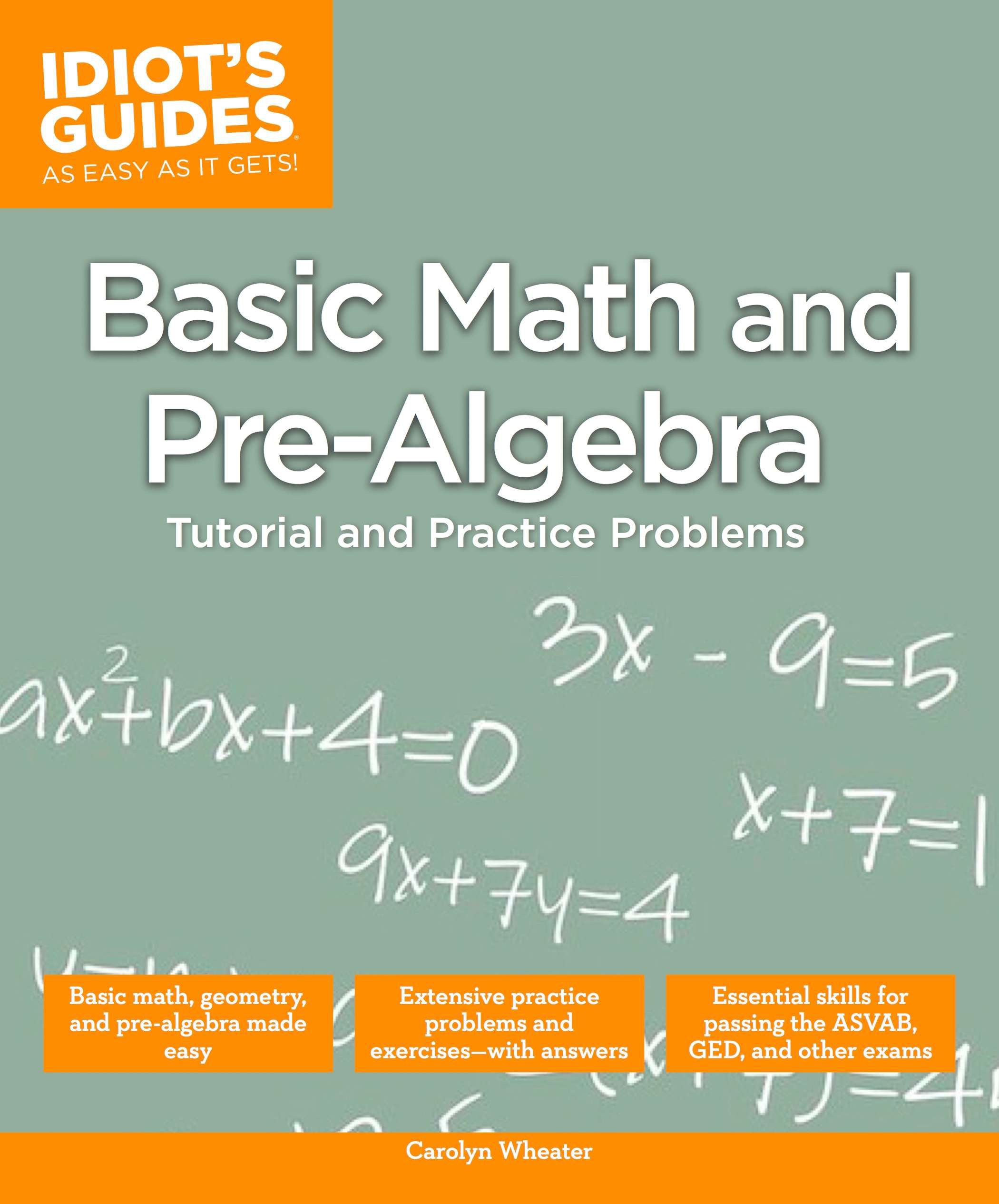Home   Algebra   Geometry   Arithmetic   Trigonometry   Probability/Statistics   Calculus   Mechanics GED   SAT   ACT   College Mathematics   GRE   School Supplies   About Us

Algebra

 Electronic TextbooksMathematics eBooksInequalities: Solve 3y + 4 > 2(y + 3) + y     3y + 4 - 4 > 2y + 6 + y - 4  (Subtracting 4 from each side.)         3y + 0 > 2y + y + 6 - 4  (Grouping like terms.)               3y > 3y + 2                  3y - 3y > 3y -3y + 2  (Subtracting 3y from both sides.)                0 > 0 + 2 Answer: Since y is eliminated, there are no             values to display. Proportions: Solve for x,  (2x + 8):(x + 2) = (2x + 5):(x + 1)     By Proportions  (2x+8)  =  (2x+5)                            (x+2)        (x+1)       (2x+8)(x+1) = (2x+5)(x+2)  (Finding the Cross Products)  2x2 + 8x + 2x + 8 = 2x2 +5x + 4x + 10  (Expanding)        2x2 + 10x + 8 = 2x2 + 9x + 10 2x2 - 2x2 + 10x - 9x = 10 - 8    (Rearranging)                           x  = 2

 Paperback Textbooks (New and Used)Functions: Given the function f(x) = 2x² - 5x + 3.  Find the values of x and draw a graph of the function.                   Let y = f(x) = 2x² - 5x + 3                      If y = f(x)               Then y = 2x² - 5x + 3                         y = (x - 1)(2x - 3) by factorization                When y = 0, x - 1 = 0 and 2x - 3 = 0                    When x - 1 = 0, x = 1                       When 2x - 3 = 0, x = 3/2 = 1½Values of x are 1 and 1½The solutions are the intercepts of the graph on the x   axis, 1 and 1½.for Math Practice,  High School  SAT  ACT

 More School Supplies and other useful items *  * *   *